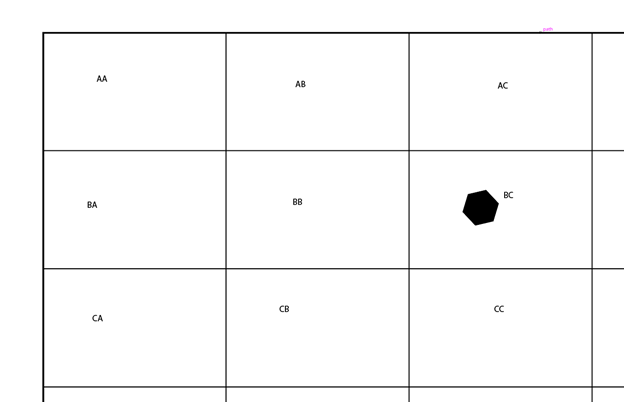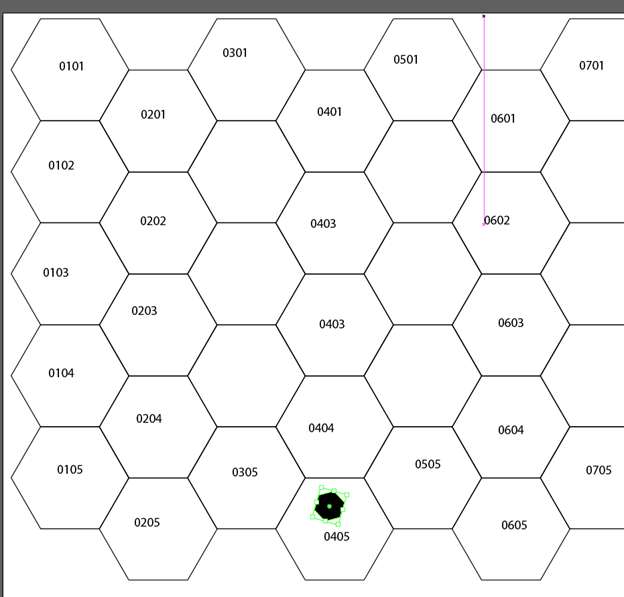# How to read map coordinates

The Hex Coordinates are simple to understand once you have the basic knowledge of their layout. The coordinates are divided into two parts, a pair of characters and then four integers. The first part represents which sub-map you are on and the second part represents which hex of that sub-map your unit is located.

#### First Part (Pair of Characters): YX XXYY

The pair of characters represent an Y and X coordinates on the map for your unit’s SubMap. The first character is the Y coordinate (A through Z) and the second character is the X Coordinate of the submap (A through Z). AA is the top left (NW) corner of the map and ZZ is the bottom right (SE) corner of the map. If the map coordinate is BC ####, then the unit is on the submap that is two submaps south and three submaps to the east on the map. See map.#### Second Part (Four Integers): YX XXYY

The four integers represent the X and Y coordinates of the unit on its submap. The first two integers form a single number (01 through 30) that represents the column (X axis) that the unit is on and the last two integers form a single number (01 through 21) that represents the row that the unit is on (Y axis). So, the layout is XXYY. If the coordinate is 0405 that means on the submap it is 4 hexes to the east and 5 hexes south. See map.Summary: This of it as a pair of coordinates, the first set of information designates the Row and Column of the submap and the second set designates the hex location the unit is at on that submap. BC 0101 means that the unit is on submap BC (Two down and three to the right) and its location on that submap is the top left corner of the submap.